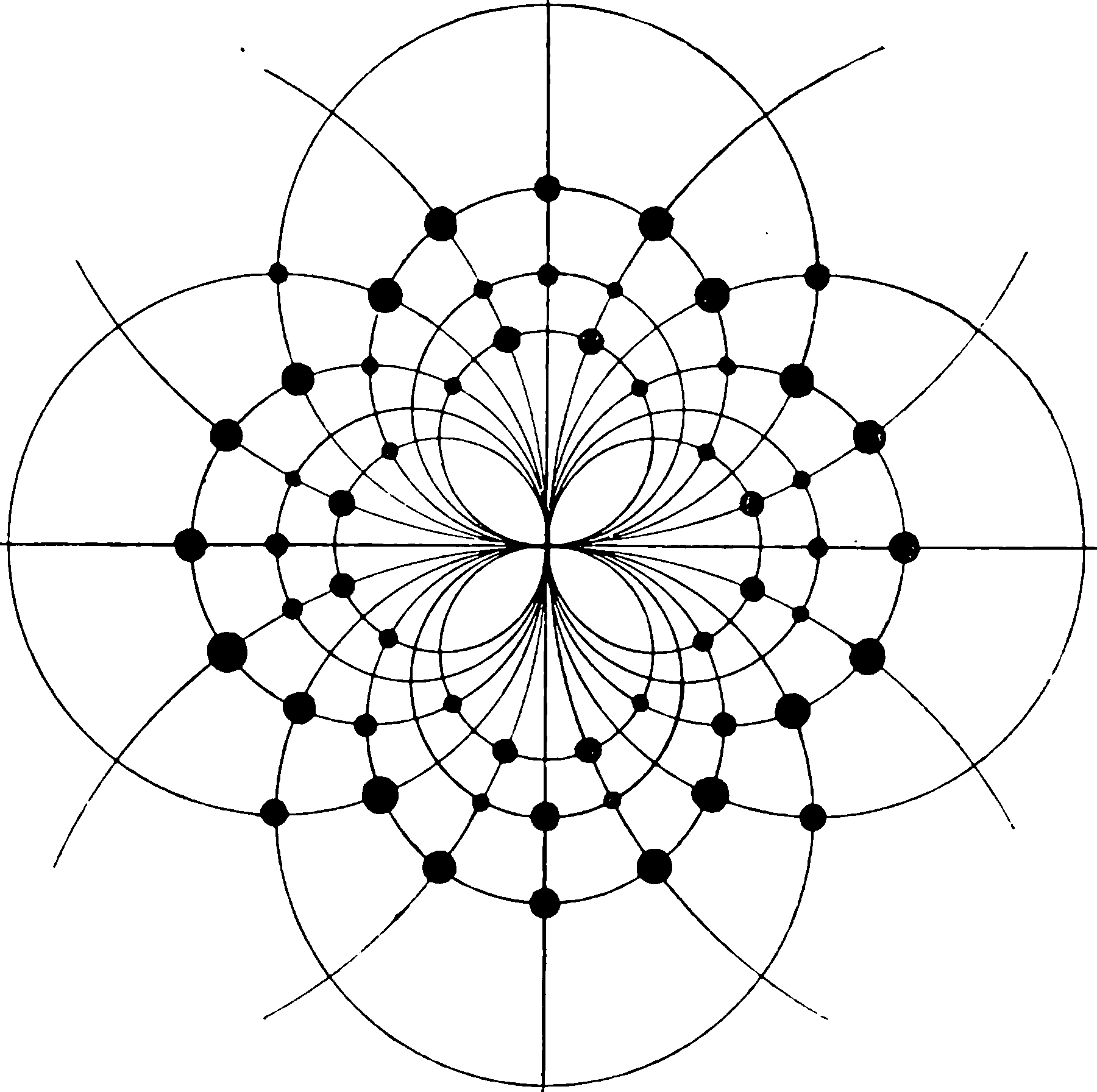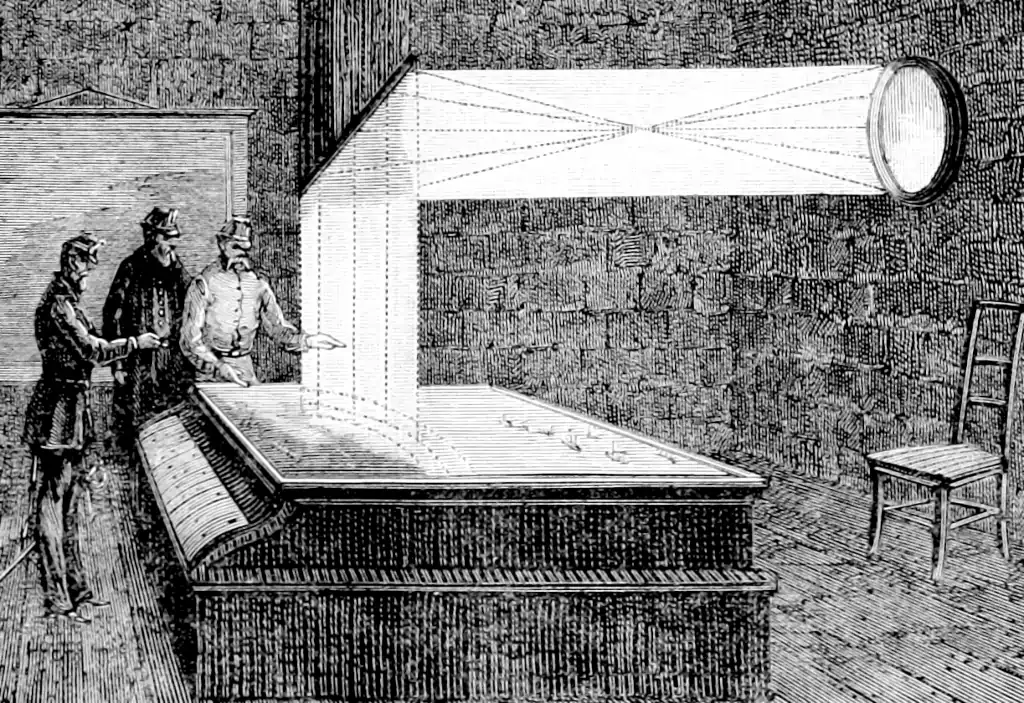# Inverse problemsX-ray crystallography is a classic artisanal inverse problem, so having a picture related to that makes me look clever.

Robert Ackroyd introduces some handy phrasing for the connections between statistical estimation theory and inverse problem solving.

Photogrammetry, MRIs, SLAM, volumetric reconstruction and X-ray crystallography are all examples of inverse problems. any of these can be constructed as classical belief propagaation, especially Gaussian BP, or in a basic case least squares.

I happen to think that this is a case where it is much easier to explain in terms of Bayesian inference, so my attempt at an actual explanation is under Bayesian inverse problems.

We can do it in terms of frequentist methods, but it does not add much in the way of explanatory value; we end up considering regularizers instead of priors, but the working in between is pretty much the same. (IMO) However, in doing so we focus on point estimates rather than entire densities, which encourages us to solve the problem by optimisation rather than integration, which is a useful insight, for example, when we consider Laplace approximations.

## Domain-specific model inversion

PEST, PEST++, and pyemu are some integrated systems for uncertainty quantification that use some weird terminology, such a FOSM (First-order-second-moment) models. They use various linear-algebra tricks to find plausible subspaces and samples.

## Interesting specific techniquesLeaning to reconstruct introduces partly-learned, partly designed reconstruction operator trick. 🏗️